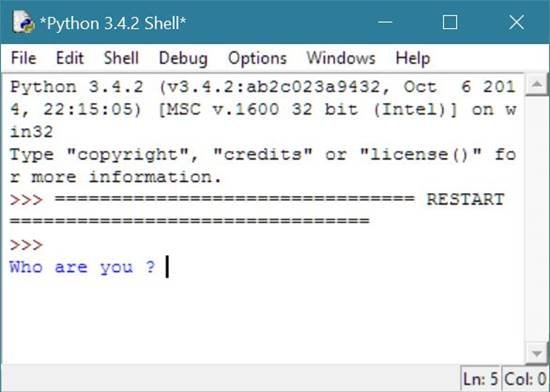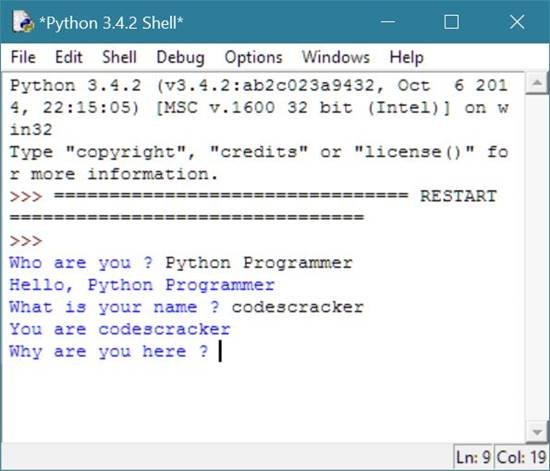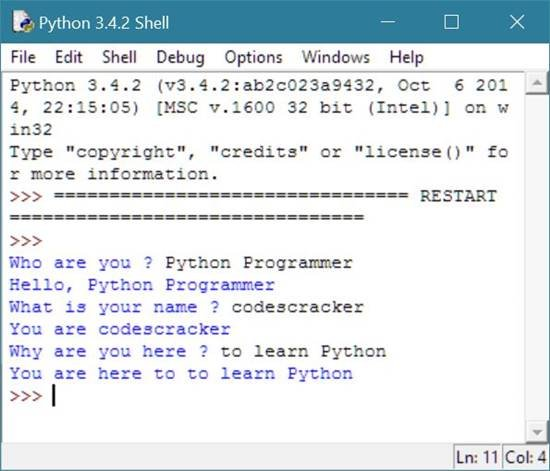# Python Variables

Variables in Python, are the reserved memory locations to store the values in a Python program. It means that, whenever you are creating a variable in Python programming, you are reserving some space in the memory for that variable.

## Create a Variable in Python

To create a variable and assign some values to it, follow the given syntax or general form in Python.

`variable_name = "value to be assign";`

You can also say that the above statement is called as assignment statement.

The above general form of creating a variable in Python, creates a variable called variable_name and assigns a value to it, the value is value to be assign.

The example given below will initialize a value Python programmer to the variable named variable_name and then print the value of the variable alone for first time and then along with Hi, for second time. Let's see how it will do this.

```# Python Variable Example
variable_name = "Python programmer";
print(variable_name);
print("Hi, "+variable_name);```

Here is the sample run of the above variable example code in Python.## Python Variables Example

Here is an example assigning values to variables in Python:

```# Python Variables - Example Program

num1=10
num2=20
sum=num1+num2

print(sum)```

Here is the sample output of the above Python program:Let's take another example on variable in Python. In this example, program will ask to the user to enter some detail to fetch the input and output accordingly as shown below.

```# Python Variable Example

variable_input = input("Who are you ? ");
print("Hello, "+variable_input);
input_variable = input("What is your name ? ");
print("You are "+input_variable);
var = input("Why are you here ? ");
print("You are here to "+var);```

Here is the sample run of the above variable example of Python.

This is the initial run or output:This is the second output after entering the detail and pressing the ENTER key:This is the third output again after entering the detail and pressing the enter button:This is the fourth output:### More Examples

Here are the list of some more examples, uses variables in python:

Tools
Calculator